# Water

Mix 21 l of water with temperature of 38 °C, 67 l warm of 34 °C and 14 l water of 32 °C.

What is the temperature of the mixed water immediately after mixing?

Result

t =  34.55 °C

#### Solution:Leave us a comment of example and its solution (i.e. if it is still somewhat unclear...):

Showing 0 comments:Be the first to comment!#### To solve this example are needed these knowledge from mathematics:

Looking for help with calculating arithmetic mean? Looking for a statistical calculator? Tip: Our volume units converter will help you with converion of volume units.

## Next similar examples:

1. NoontimeIn the morning temperature was 110°F. By noontime it has gone up by 15°F. What was the noon temperature. (Give your answer in °F)
2. Temperature changeThe mean temperature change is -3.2°F per day for five days. What is the total change over the five day period?
3. OpheliaOphelia recorded the temperature of a cold store every two hours. 1. At 6am it was -4°C and at 8am it was -1°C. By how much did the temperature rise? 2. The temperature went up by 5°C in the next two hours. .What was the temperature at 10am?
4. Without 2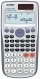Without multipying, tell whether the product 0.644 x 0.25 will be greater than 1 or less than 1? Explane how you know. Then find the product.
5. Average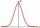Average of 7 numbers is 65. What is its sum?
6. Double 5Peter was thinking of a number. Peter doubles it and gets an answer of 8.6. What was the original number?
7. Division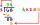Divide by the number 0.2 is the same as multiply by what number?
8. Hexadecimal number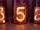What will be hexadecimal number 303 as decimal number?
9. Quotient 3If the quotient of 8/13 and 2 is subtracted from the product of 1 3/4 and 8/21, what is the difference?
10. AverageThe arithmetic mean of the two numbers is 71.7. One number is 5. Calculate the second number.
11. EvaluateThe division of numbers 18 and 6 increase by product of the numbers 156 and 0.001
12. Combine / add termCombine like terms 4c+c-7c
13. Evaluate expressionEvaluate expression using BODMAS rule: 1 1/4+1 1/5÷3/5-5/8
14. Farmer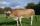The farmer farming 293 ha of arable land and the remaining 20% are meadows and pastures. How much land farmer owns?
15. Ratio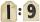Unfold number 1963 in the ratio 9:2:2.
16. Summerjobs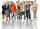The agency give summerjobs for 2352 students in 2018. The eighth was high-school students, the rest was undergraduates. How many undergraduates works via agency in 2018?
17. Juice box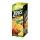In the box is 0.3 liters of juice. How many liters of juice contains 3 these boxes?